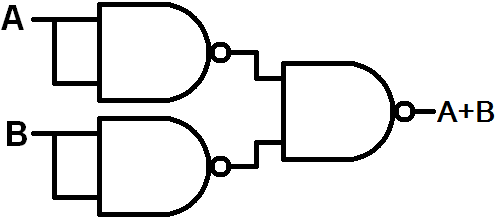Write the truth table of the OR gate and the NAND gate. How the OR gate is drawn by using the NAND gate?

Considering, 1 as high input/output and 0 as low input/output

OR Gate (Truth Table)

 First  Input(A) Second Input(B) Output(Y) 1 1 1 1 0 1 0 1 1 0 0 0

NAND Gate (Truth Table)

 First Input(A) Second Input(B) A.B(AND output) NAND Output(Y) 1 1 1 0 0 1 0 1 0 0 0 1 1 0 0 1As shown in the above figure, three NAND gates are connected such that the output of first two NAND gates are connected as inputs of the third NAND gate. The inputs for first two gates are combined to behave as a NOT gate. Therefore, these compliment outputs serve as input for the third NAND gate and an OR gate is obtained as shown by truth table of NAND gate.

TRUTH TABLE

 First gate input(A) Second Gate Input(B) First Input of third gate Second Input of Third Gate Output of third gate(Y) 0 0 1 1 0 1 0 0 1 1 0 1 1 0 1 1 1 0 0 1